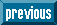## Indicators

•Cited by SciELO
•Access statistics

## Related links

•Similars in SciELO

## On-line version ISSN 2007-9869Print version ISSN 1870-6622

### EconoQuantum vol.11 n.2 Zapopan Jul./Dec. 2014

Suplemento

Assigning a value difference function for group decision making

Laura Plazola Zamora1, Salvador Sandoval Bravo2, Jaime Mora Vargas3

1 Universidad de Guadalajara, Periférico Norte 799. E-mail: azucenadelrey@yahoo.com.mx.

2 Universidad de Guadalajara, Periférico Norte 799. E-mail: salvsanb@cucea.udg.mx.

3 Tecnológico de Monterrey, Campus Estado de México. E-mail: jmora@itesm.mx.

Recepción: 15/01/2014.
Aceptación: 01/06/2014.

Abstract

In this paper we propose a procedure to determine an individual preference aggregation. The procedure is based on the concept of second order preferences. If the preference strength of each group member can be modeled with an additive value difference function, then the influence of each individual in the decision of the group is approximately proportional to the value difference between the best and the worst alternative.

The problem of finding a value difference function, which represents a known preference of the possible rankings of the set of alternatives, is solved as a linear programming problem. An implementation of the procedure has been developed with the Delphi programming environment.

Keywords: group decision, value difference function, additive value function, decision support system.

Jel Code: D70, D71, C44.

Resumen

En este trabajo se propone un procedimiento de agregación de preferencias individuales, basado en el concepto de preferencias de segundo orden. Si la intensidad de la preferencia de cada miembro del grupo puede modelarse con una función de diferencia de valor aditivo, entonces la influencia de cada individuo en la decisión del grupo es aproximadamente proporcional al valor de la diferencia entre la mejor y la peor alternativa.

El problema de encontrar una función de diferencia de valor que representa una preferencia conocida de los posibles ordenamientos del conjunto de alternativas se resuelve como un problema de programación lineal. Una aplicación del procedimiento ha sido desarrollada con el entorno de programación Delphi.

• Introduction

Groups such as directive committees, administrative teams, legislative bodies and society itself, are frequently faced with the responsibility of making decisions and, although the members of the group may have a common interest, they could differ with regard to their opinions and preferences. This situation renders group decision making a very complex process. Formally, group decision making refers to the practice of obtaining a group preference on a given finite set of alternatives, starting from individual preferences through a process called constitution.

The formal study of group decision making began more than two centuries ago with Jean-Charles de Borda and the Marquis de Condorcet, but the theory adopted its modern form with Arrow's Impossibility Theorem (1963). Arrow proposed a set of intuitively satisfactory axioms that every aggregation procedure of individual preferences be fulfilled (Blair and Pollack, 1983). In this result it is affirmed that for each possible constitution there is at least one set of possible individual preferences that the group ranking construction violates at least one of the axioms. Each possible constitution is potentially unfair or irrational (French, 1988).

A considerable amount of literature has been produced after Arrow's famous theorem; several paths have been explored in trying to avoid the impossibility. In some cases, restrictions have been suggested for the profiles of admissible individual preferences (Black, 1958; Maskin, 1995; Massó, 1996; Schwartz, 2001); and in some other, the transitivity condition has been substituted for a weaker property, such as quasi-transitivity or acyclicity (Mas-Colell and Sonnenschein, 1972; Peris and Sánchez, 2001). The first case is of interest only in some particular cases and, although specific circumstances make it possible to escape of the impossibility, there is no appropriate procedure to establish group value rules in an efficiently enough, rational, or democratic way (Villar 1988). In the second case it was found that there were no satisfactory constitutions, since all quasi-transitive group decision functions imply the existence of an oligarchy. In the same way, under certain additional conditions, the constitution was dictatorial (Mas-Colell and Sonnenschein, 1972).

There is a third approach concluding that a group aggregation rule guaranteeing, in all the situations, that the decisions can be rational and democratic, requires not only taking into account the group members' preferences, but also the strength of their preferences. In Arrow's formulation, both the individual and the group rankings do not consider individuals' preference strength.

This last approach also runs into impossibility: the one of making comparisons of preference strength among different individuals. Measurement and interpersonal comparability of utilities have been investigated by Roberts (1979), Blackorby et al. (1984), D'Aspremont and Gevers (2001), who have proved that the introduction of such concepts makes it possible to find aggregation procedures that satisfy similar conditions to Arrow's conditions. Unfortunately, an objective method to make interpersonal comparisons has not yet been developed, and there is no way of satisfying the conditions of equity and rationality unless those comparisons can be made.

The lack of information has motivated the search for new aggregation methods that incorporate magnitudes reflecting the individual's influence on group decisions. Arora and Allenby (1999) developed a hierarchical Bayesian group decision model that yields individual estimates of influence at the product attribute level. The proposed model relates the measures of influence for identifying individuals to high influence on group decision. Jabeur and Martel (2002) have proposed a method for quantifying the relative importance of the members of the group for every pair of actions. Other works related to group decision making considering preference strength are Dyer and Sarin (1979), Cook and Kress (1985), Wakker (1998), Kim and Ahn (1997), Harvey (1999), Köbberling (2006) and Cato (2014).

A path scarcely explored is the one of adding preferential information resulting not from the comparison of the preference strength among group individuals, but from having equitably taken into account the preferences of the group members, establishing the contribution of each one of them to the group ranking, and trying to have everyone equally influencing the final decision.

This paper incorporates a criterion of equity among individuals, in which everybody influences the group ranking to the same degree. In order to achieve this, it is necessary that preferential information of the individuals not only includes a ranking of the alternatives, but also data on the strength of their preferences. According to Sen (1974; 1976) the use of meta-rankings (orderings of the rankings of the alternatives) in the problem of social choice can be applied to the problem of finding a meaningful measure of cardinal utility. Preferential information on the influence that the group members have on the collective decision can be obtained by means of the concept of second order preferences, which considers the preference of each group member over the set of rankings on A, interpreted as possible outcomes of constitution rule's application.

If the preference strength of each group member can be modeled with an additive value difference function, then the influence of each individual in the decision of the group is approximately proportional to the value difference between the best and the worst alternative, which suggests that the constitution must provide the adjustments necessary for this difference to be the same for each group member.

• Second order constitution

This work focuses on an additive function4 constitution in which each individual iI of the group expresses his evaluation function in a closed, bounded and non-empty subset Y of real numbers common to all members of the group, vi: AY; the preference of the group pg is given by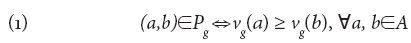where vg is given by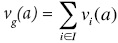(French, 1988; Keeney, 1976).

A second order constitution takes into account the preference of each member i of the group over the set of weak orders on A, interpreted as possible results Pg of the group choice. To represent these preferences we take a reference set, common to all members of the group, denoted as O(A), and fashioned by all the possible rankings of the set A in decreasing preference order, each one with the form a1a2...am. In such way, each member i of the group has an associated binary relationover O(A), in regard to which Pi will be strictly the best element of O(A), in the case where it does not include indifferences, for the case where indifferences exists, then Pi is represented by a subset of O(A). In order to be more precise, relationtotally determines Pi, as an element or subset of O(A), according to the case by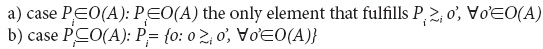Pi as weak order over A is called first option of the individual i over O(A). This weak order is in the first case the elementitself, and in the second one the transitive closure of the subset. Therefore, the set of preferences over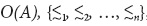, called second order preferences profile (of the group) on A, determines univocally the first order preference profileon A.

A constitution is a second order constitution when the preferential information processed for each member i of the group determines univocally a weak order, over O(A), in such a way that the respective preferencesover O(A) and the group choice Pg fulfill the following condition:

Pareto Optimality of the Pg with respect to the second order preferences profile: if the choice of the group PgO(A),(or any element PgO(A)) is substituted by another element belonging to O(A), then, at least one member i of the group loses strictly in the sense of his relationover O(A).

The preference of the group on A, given by (1) can be expressed aswhere I(a,b)I is the set of group members that prefer a to b,The preference of the group on A (2) admits an interpretation similar to simple majority rule, just that instead of comparing the cardinality of sets I(a,b) and I(b,a) the comparison is among the respective sums of preference strength: for the individuals in I(a,b) class, differences vi(a)-vi(b) are added; and for I(b,a) class, differences vi(b)-vi(a) are added. The group preferences between options a and b is set to be that of the class with larger results. Then every individual that prefers a over b contributes not with a vote but with a real number vi(a)-vi(b), which is called "vote magnitude" of individual i. The preference of the group for each alternative pair a, b, on the choice set {(a,b), (b,a)}, is given by the ordinal value function with two arguments wg(⋅,⋅),Since we are considering I(a,b) and I(b,a) as non-empty sets, i.e. it is excluded the situation where all group members have the same preferences regarding two alternatives, then we define wg(a,b)=wg(b,a)=0.

A class A constitution (of additive function) implicitly contains a voting system that, in addition to the aforementioned choice sets with the form {(a,b), (b,a)}, includes the choice set O(A). The magnitude of the vote wi(o) of the individual i for the element oO(A) to be selected as ranking of the group is equal to the sum of the magnitudes of votes that the individual i assigns to each one of the ordered pairs belonging to such element, (a,b)o, that is,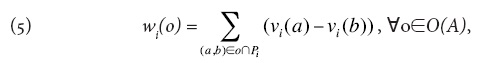where Pi is the weak order on A associated to the ordinal value function vi, given byAccording to the aforementioned, in a class A constitution, each evaluation function vi determines a preference Pi on A given by (6) and a preferenceover O(A) given byWe can then say that(Pi) is the revealed preference represented by vi on O(A) (on A, respectively).

A binary relationon O(A) agrees with a value difference function on A, if there is a real function vi on A such that (7), in which case we can say thatagrees with the (value difference) function vi, and vi is a value difference function over A that represents. Here it is worth mentioning that "value difference function" is referred to any function that represents the preference of an individual over O(A).

Before the following theorem, which gives sense to the previous definitions, it is convenient to make explicit the properties of the revealed preference on O(A).

Lemma 1. In a class A Constitution every preferenceon O(A) holds:

a)is a weak order on O(A) which ordinal value function is wi,

b) The first option ofon O(A) is the weak order Pi on A given by (5). Furthermorec)is invariant under affine transformations of vi.

Proof a) Obvious of (7). b) directly of (5) results (8); Pi maximizes wi(·), therefore is the first option ofon O(A), because the summation in (8) includes every pair (a,b) in which vi(a)> vi(b), c) Immediately.

Theorem 1. In a class A constitution:

a) The weak order of the group, Pg, is the transitive closure of the elements in O(A) that maximize the function wg on O(A), given bywhere the wi are given by (5), fulfillingb) The Pareto optimality condition is satisfied: the choice of the group Pg is a Pareto optimum with respect to the revealed preferenceson O(A).

c) Arrow's conditions hold.

d) If the evaluation functions of every group member suffer positive affine transformations, then, for the inequalitiesas for the ranking Pg of the group and for the preference relationof the group over O(A) to remain unchanged, it is required that such affine transformations are identical.

Proof a) Let substitute (5) in (9), we obtain (10):By (2) the value of wg(·) over O(A) given by (10) is maximum for a certain element oO(A) if and only if (a,b)o always that vg(a)>vg(b); o equals to PgO(A) or is an element from PgO(A); then Pg is the minor week order that contains all elements from O(A) that maximizes the function wg(·).

b) It is sufficient to demonstrate by contradiction that for each oO(A) different of Pg (or not in Pg), exist at least a group member i such that Pgo. Let consider the case PgO(A) and let be oO(A) such that oPg for all i, where oPg for some i. Then by (7),Some of the inequalities are strict. Let sum orderly to both sides of the inequality for all iI,That is to say,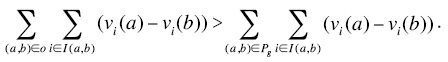By (10) this last inequality is equivalent to wg(o) > wg(Pg), which is a contradiction of a). The proof of the case Pg O(A) is similar, just substitute Pg by o'Pg.

c) See Keeney (1976) and French (1988). d) It is demonstrated directly making the corresponding substitutions.

Since the relationcontains less preferential information than the function vi, it is natural to first try to determine the preferenceon O(A), by a value difference function, and then select the evaluation function vi that will be declared to the group within the evaluation functionsset compatible with, given byThe evaluation functions in this set are equivalent in the sense that they equally represent the preferenceover O(A), with no positive affine transformation relating both of them.

• Assigning a value difference function

Given the Theorem 1, for each member of the group, subject to a class A constitution, in complete ignorance conditions over the preferences of the other members, it is convenient to express a value difference function v on A (here the subscripts of the group members are omitted) representing, as best as possible, his preferenceover O(A), Therefore, the following relation is fulfilledwhere P is the weak order on A corresponding to his preference over A, given byand (a,b)o-P denotes the alternative pairs that are in o but not in P. Therefore, the terms in both summations in (13) are all non-negatives, and each one of them corresponds to a pair in o with opposite sense to P.

Relation (13) is equivalent to relation (7) when the subscript i is eliminated, due to the fact that the right side of the equalityis a constant not depending on oO(A) (equal to the magnitude of the vote w(P) for the first option P with respect to. Relation (13) is more appropriate than (7) for the individual problem of determining a value difference function. Here the ranking of the elements of O(A) is not based on the magnitude of the vote (in favor), as it is in (7), but it is given instead in terms of the magnitude of the votes against, or the cost c(o), given by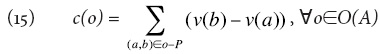therefore, (13) can be rewritten as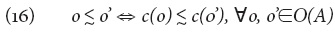Let be s = (sm, sm-11,..., s1)O(A) a first option ofover O(A), which is taken as reference and hence called seed. Therefore s = P, if P does not contain any indifference over A and, in the opposite case, sP. Let be Kj(o) the amount of times that the consecutive alternative pair in s, (sj+1,sj) appear directly or indirectly in opposite order to o,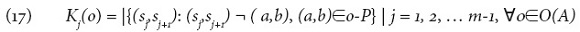being |.| the cardinality function and ¬ the inclusion relation given by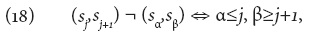Let be CO(A) the set of all rankings ojO(A) obtained by the exchange of two consecutive elements, sj+1,sj, in s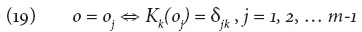where δjk is the Kronecker delta. By (15)and by (16)The problem of finding a value difference function representing a known preference on O(A), which agrees with a value difference function on A, can be solved by (22), as a linear programming problem (Krantz et al., 1971), in which each equality caused by an indifference is used to eliminate the unknown quantity c(oj), and each inequality corresponds to the difference of cost of an element oO(A) and its immediate successor o'O(A), resulting in a linear problem of the form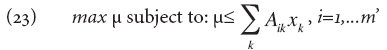where each xk corresponds to c(oj). The corresponding value difference function is determined by giving an arbitrary value to v(si), o for instance; the rest of the values of the function are given bySince it is assumed thatagrees with a value difference function, it is easier in practice to determine simultaneously such preference and a value difference function v on O(A) representing it.

Once that each group member has solved the problem of finding the value difference that represents his preference over AxA, remains to solve the controversial problem of assigning scale and origin to each one of those functions. For the additive case, in which this work regards, the origin of value differences functions does not affect group choice, then the problem is reduced to assignment of quantities:where Mi represents the scale of function vi and the preference strength between first and last option over A for individual i. In other words, all interpersonal comparisons of preference strength that could emerge for the group choice, which has the form "the preference strength of a over b for individual i is bigger than the preference strength of c over d for individual j", represented by vi(a) - vi(b) > vj(c) - vj(d), are formally solved by the assignment of preference strength Mi between the first and last option over A for each individual i.

Our proposal does not try to solve the problem of interpersonal comparison in a direct way, but indirectly with base on that all members should have the same influence in the group decision, the magnitudes Mir reflect in some way such influences. According to this, we suppose that an argumentation similar to the well-known Laplace insufficient reason principle, developed in the context of a priori probabilities assignment, could convince all group members that this values should be equals, unless that exist enough reasons to make the difference between Mi, without discrimination of group members or alternatives. Such reasons could work to adjust Mi according to an invariant correction scale factor that faces arbitrary changes in assignment of alternatives and designation of group members.

The preference strength of individual i can be displayed on a line segment with lengthwhose two edges represent the best and worse alternatives of A for each group member i called extreme alternatives of i. For these two edges each of the alternatives is represented within the segment according to its coordinate vi, resulting that the distance for any pair of alternatives is proportional to the respective preference strength. These scales are not comparable for different individuals because the "preference strength" Mi between the extreme alternatives of member i is a quantity that the group assigned to individual i (although it is the same for all group members), which relates to the relative influence of the individual in the group's decision and not to their preferences on the set A, so it is a magnitude of reference against the other preference strengths of individual i are measured. Therefore, interpersonal comparisons of preference strengths are not absolute, they depend on the presence in A of alternatives, namely the extreme alternatives of both members of the group. That is why subsection d) of the previous theorem states that in class A constitutions interpersonal comparison of the preference strength between two members i and j of the group, given by (10), is maintained only if all the functions of evaluation transform under the same positive affine transformation.

The key idea is to model, through a difference value function vi, the rankings on O(A) for each individual i, called second order preferences. We developed a computer program in which each individual graphically specifies the strength of his preferences among the alternatives in A, then the program generates all possible permutations from the initial ranking. With this information our model determines a first version of vi. In order to select better functions vi modeling the ranking on O(A) for each individual i, we solve a linear program which maximizes the minimal difference between consecutive elements in the ranking on O(A). In this way, the constitution provides the necessary adjustments for this difference to be the same for each group member.

• Software development

A computer program, PRESEO, was designed in Delphi 7 to solve the group decision problem with second order preferences. At the present time, the software solves problems of up to five alternatives. The menu on the main interface indicates the six steps required to complete the procedure.

As a proof-of-concept, let us consider the following scenario: three individuals deciding on a set of three alternatives a, b, c, hold the following strict preferences:Using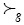to indicate the strict preference for the group, the simple majority rule leads to a cycle, and the preference of the group shows a non-transitive result since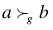, because two out of the three individuals prefer a to b; and alsobecause two out of the three prefer b to c; finallyfor the same reason as above. In this case, the only information available is the alternative rankings of each individual, which means that we only have first order information.

Considering the concept of second order preferences, each member of the group establishes a weak order as first option; the remaining rankings are the result of compromising their "less strong" preferences. In this way, each individual must rank the alternatives according to his preferences using the buttons "Más" (more) and "Menos" (less) according to their preference intensity. Figure 1 presents a screenshot from PRESEO, where the ranking of the alternatives for one of the participants have been indicated. Notice the proximity between alternatives a and b, compared to that for alternatives a and c. The screenshot above shows the case with 3 alternatives, where only one alternative is needed to locate (since one of the alternatives is the highest and the other the lowest), for that remaining alternative his preference will be determined by its position in the scale.

Our approach starts from ordinal information and then cardinal information is used a priori, in order to obtain the order for rankings of the alternatives to calculate differences among consecutive rankings evaluations. A procedure similar to MACBETH method (Bana e Costa, Vansnick 1994, 1997) is used to estimate value difference functions in the context of individual multicriteria decision making.

Finding a value difference function that can represent the individual preferences over O(A) arises as a problem. The procedure to determine a consistent value function in conformity with the ranking is shown next.

Sketch of the method

1. Generate the permutations O(A) from the initial ranking, constituting the second order preferences. Notice that generation of all possible permutations O(A) could require major computer resources depending on the number of alternatives and individuals.

2. Calculate the magnitudes of the votes against for each permutation with the expression.

3. Estimate the differences between the consecutive magnitudes of the vote. Here the results are several algebraic expressions that will form the right sides of the restrictions of the linear program to be solved.

4. Solve the linear problem.

5. Substitute the value found in the magnitudes of the vote against.

6. Aggregation step: once the magnitudes of the vote against have been estimated for each individual, we shall proceed to add the group information by means of the expression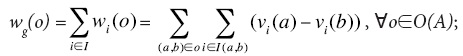The "winner ranking" will be that with the smallest magnitude of votes against. For example, table 1 below shows second order preferences for individual 3 and the corresponding votes against. We know that v(b)=0, v(c)=10, and we want to obtain v(a), let us say that v(a)=x, that is on the bar grading from 0 to 10.We know that his first order ranking is cab, its magnitude of the vote against is zero because individual 3 does not "sacrifices" anything respect his first order ranking; in fact, it is his first order ranking. But if we consider the ranking cba and we compare with the first order ranking, there is an interchange between a and b, and calculating the vote against we obtain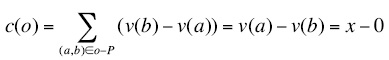In the same way for acb, he did an interchange between a and c, thenand so on for each second order preference. We have to recall that x is unknown and represents v(a), the value that we want to determine. Once calculated all the votes against, we calculate the differences between consecutive evaluations:Then, we propose the following linear programor, equivalentlywhere μ=min{[x],[-2x+10],[2x]}. Based on this procedure that "minimizes errors" we will know, only with ordinal and preference strength information, the valuations of each individual. The optimal solution is x=3.33 (the aimed value function). Table 3 shows the preferences over the set O(A) for a value of x equal to 3.33,

The procedure is the same for each individual. Group ranking is obtained by summing up the votes against for all the elements in O(A) for each individual in the group. The following screen gathers information on every individual partaking in the decision and the magnitude of the group vote is calculated for each ranking (figure 2). A table with the value functions for each alternative and each individual is shown including the resulting social order. Note that the group ranking is not a cycle. There is a tie between the rankings acb and cab, because their magnitudes of the votes against are exactly the same.

Example5

Consider the following problem: The board of the department of Quantitative Methods, belonging to the Center of Economic and Administrative Sciences of the University of Guadalajara, has to form a committee responsible for financial support decisions. The committee has five positions available: president, secretary, spokeperson, first substitute and second substitute. The departmental board is set up by the head of the department (jefe), the academy presidents (there are four academies: Mathematics (Mate), Optimization (Opti), Statistics (Est.) and Mathematical Economy and Econometrics (Eco) and the Head of the Economic Theory Research Center (CITEC). Then there are six individuals who make the decision over a set of five alternatives (teachers): {P1, P2, P3, P4, P5} to be distributed in the five positions available (president, secretary, spokeperson, first substitute and second substitute). In that sense, an assignment (P5, P3, P2, P1, P4) states that teacher P5 will serve as president, teacher P3 will be secretary, P2 will be spokeperson and P1 and P4 teachers will be first and second substitutes, respectively.

The screens with the results thrown by PRESEO are shown next. Figure 3 shows the data menu.

Individual rankings of preferences are:Each individual has to express an order of preferences. The preference strengths are given by the position of the alternatives on the scroll bars. Most preferred alternative is closer to ten. Figure 4 below shows the individual preferences and the corresponding preference strength for the head of the department:

In this case there are five alternatives leading to 5 = 120 possible rankings, but the decision maker does not need to compare all rankings on O(A), since, as aforementioned, the software calculates the magnitudes of the votes against for each permutation, estimates the differences between the consecutive magnitudes of the votes against, generates and solves the linear program and, once the magnitudes of the votes against has been estimated for each individual, it aggregates the information to obtain the group ranking.

After that, we obtain the corresponding value functions, also the vote magnitudes for each alternative. Then, the group rankingis obtained in order to determine the committee members. Thus, P2 is a first ranked teacher at position of president; second ranked teacher (P4) in the position of secretary and so on. Figure 5 presents screenshot from PRESEO with the information mentioned above.

Ideally, after obtaining the order of the group, members should discuss and reach consensus, this part is not yet implemented into the software. This paper shows how does the method work and how it was implemented into a computational application.

• Concluding remarks

In the present paper we propose an individual preference aggregation procedure based on the concept of second order preferences. If the preference strength of each group member can be modeled with an additive value difference function, then the influence of each individual in the decision of the group is approximately proportional to the value difference between the best and the worst alternative.

An algorithm capable of assigning a value function on a finite set of alternatives has been developed for each individual in the problem of group decisions with second order preferences, using ordinal information and information about the preference strength of the individuals. In order to find a value difference function representing a known preference of the possible rankings of the set of alternatives, a linear programming problem is generated and solved.

The problem of interpersonal comparison is avoided by the assumption that every group member has the same influence on group decision.

Up to now, the software considers only strict preferences; nevertheless, it is necessary to put the procedure into practice in situations involving indifference. In the same way, the software developed only solves problems of up to five alternatives, but we are trying to extend this number. Its main drawback at present is that the O (A) set is extremely large, and calculation tasks turn out to be very exhaustive. We are also working on a web version of the software to develop online applications for real decision-making problems.

An advantage of our proposed approach is that the traditional methods to build a value difference function require that the preference strength over the set of alternatives represent a difference measure structure (French, 1988, Krantz et al,. 1971, Roberts, 1979), which includes a solubility condition hard to achieve in practical group decisions problems. Our proposal does not require hypothetical alternatives in order to fulfill the solubility condition. Another advantage in our approach is the consideration of an equity criterion, meaning that all individuals have the same influence or "number of votes" in the ranking of the group.

Conditions about solution uniqueness in (23) require further investigation and will be the subject of future work.

The problem of finding a preferenceon O(A) agreeing with a value difference function is formally equivalent to the problem of assigning a probability measure on a finite set (Fishburn et al., 1989; Roberts, 1979), and both problems are equivalent to the assignment problem of the scaling constants ki.

• References

Arora,N; Allenby, G. M. (1999). Measuring the Influence of Individual Preference Structures in Group Decision Making. Journal of Marketing Research, 36 (4): 476-487.         [ Links ]

Arrow, K. (1963). Social Choice and Individual Values 2nd Edition. New York: John Wiley &         [ Links ] Sons.

Bana e Costa, C. A.; Vansnick, J. C. (1994). MACBETH-An Interactive Path Towards the Construction of Cardinal Value Functions. International Transactions in Operations Research 1 (4): 489-500.         [ Links ]

Bana e Costa, C. A.; Vansnick, J. C. (1997). Applications of the MACBETH Approach in the Framework of an Additive Aggregation Model. Journal of Multi Criteria Decisions Analysis, 6 (2): 107-114.         [ Links ]

Black, D. (1958). The Theory of Committees and Elections. Cambridge: Cambridge University Press.         [ Links ]

Blair, D.; Pollack, R. (1983). Decisiones Racionales Colectivas. Investigación y Ciencia. Investigación y Ciencia, octubre 1983, pp. 64—72.         [ Links ]

Blackorby, C. Donaldson, D.; Weymark, J. (1984). Social Choice with Interpersonal Utility Comparisons: A Diagrammatic Introduction. International Economic Review, 25 (2): 327-356.         [ Links ]

Cato, S. (2014). Menu Dependence and Group Decision Making. Group Decision and Negotiation, 23 (3): 561-577.         [ Links ]

Cook, W.; Kress, M. (1985). Ordinal Ranking with Intensity of Preference. Management Science, 31 (1): 26-32.         [ Links ]

D'Aspremont, C.; Gevers, L. (2001). Social Welfare Functional and Interpersonal Comparability. CORE discussion paper 2001/40. Université Catholique de Louvain, Belgium.         [ Links ]

Dyer, J.; Sarin, R. (1979). Group Preference Aggregation Rules Based on Strength of Preference. Management Science, 25 (9): 822-832.         [ Links ]

French, S. (1988). Decision Theory: An Introduction to the Mathematics or Rationality. John Wiley & Sons. New York.         [ Links ]

Harvey, C. M. 1999. Aggregation of Individuals' Preference Intensities into Social Preference Intensity. Social Choice and Welfare, 16: 65-79.         [ Links ]

Keeney, R. L. (1976). A Group Preference Axiomatisation with Cardinal Utility. Management Science, 23 (2): 140-145.         [ Links ]

Kim, S.H.; Ahn, B. S. (1997). Group Decision Making Procedure Considering Preference Strength Under Incomplete Information. Computers and Operations Research, 24 (12): 110-1112.         [ Links ]

Köbberling, V. (2006). Strength of Preference and Cardinal Utility. Economic Theory, 27 (2): 375-391.         [ Links ]

Krantz, D. H.; Luce, R. D.; Suppes, P.; Tversky, A. (1971). Foundations of Measurement. Vol. 1. New York: Academic Press.         [ Links ]

Fishburn, P. C.; Roberts, F. S. (1989). Axioms for Unique Subjective Probability on Finite Sets. Journal of Mathematical Psychology, 33 (2): 117-130.         [ Links ]

Jabeur, K.; Martel, J. M. (2002). Quantification de l'importance relative des membres d'un groupe en vue de déterminer un préordre collectif. INFOR Journal, 40 (3): 181-197.         [ Links ]

Mas-Colell, A.; Sonnenschein, H. (1972). General Possibility Theorems for Group Decisions. Review of Economic Studies, 39 (2): 185-192.         [ Links ]

Maskin, E. (1995). Majority Rule, Social Welfare Functions, and Game Forms. In Basu K, Pattanaik P, Suzumura K. 1995, Choice, Welfare, and Development: A Festschrift in honour of Amartya K. Sen. Oxford & N. York. Clarendon Press. Pp. 100-109.         [ Links ]

Massó, J. (1996). La teoría de la elección social: Métodos de votación no manipulables. Universitat Autònoma de Barcelona: Mimeo.         [ Links ]

Peris, J.E.; Sánchez, C. (2001). Characterization of Social Choice Sets in Terms of Individuals' Maximal Sets: The Fixed Agenda Framework. Social Choice and Welfare, 18: 113-127.         [ Links ]

Roberts, F. S. (1979). Measurement Theory. Addison New York: Wesley.         [ Links ]

Salo, A. (1995). Interactive Decision Aiding for Group Decision Support. European Journal of Operational Research, 84 (1): 134-149.         [ Links ]

Salo, A.; Punkka, A. (2005). Rank Inclusion in Criteria Hierarchies. European Journal of Operational Research, 163 (2): 338-356.         [ Links ]

Schwartz, T. (2001). From Arrow to Cycles, Inestability, and Chaos by Untying Alternatives. Social Choice and Welfare, 18: 1-22.         [ Links ]

Sen, A. (1974). Choice, Orderings and Morality. In Choice, Welfare and Measurement. (1997). Harvard MA: Harvard University Press. Pp. 74-83.         [ Links ]

Sen, A. (1995). Rational Fools: A Critique of the Behavioural Foundations of Economic Theory. In Nueva economía del bienestar. Universitat de València.         [ Links ]

Villar, A. (1988). La lógica de la elección social: Una revisión de los resultados básicos. Investigaciones Económicas, Segunda Época, 12 (1): 3-44.         [ Links ]

Wakker, P. (1988). Derived Strengths of Preference Relations on Coordinates. Economics Letters, 28 (4): 301-306.         [ Links ]

Notas

4 Some important results about the use of additive value functions in group decision making are in Salo and Punkka (2005), Salo (1995).

5 The example is fictitious, only used to show the methodology to the departmental board.All the contents of this journal, except where otherwise noted, is licensed under a Creative Commons Attribution License